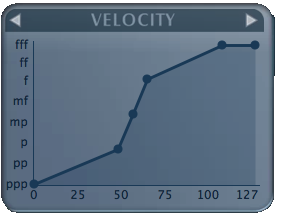## Remapping Keyboard Velocity

19 Apr 2014

As a musician, one of the first things I need to address is the 'feel' of my instruments. I'm sure that down the line, there will be a sweet device for this sort of transformation, but for now, we can do it entirely within Bitwig API.

Revisiting Generic Keyboard Implementation, we have `host.getMidiInPort(0).createNoteInput("MIDI Keyboard");` inside `init()`. This function returns an object of type `NoteInput` which has the method `setVelocityTranslationTable()`.

``````var noteInput = host.getMidiInPort(0).createNoteInput("MIDI Keyboard");
noteInput.setVelocityTranslationTable( table );
``````

This method takes an 128 length array, or a hash table, transforming the index of the array into the values of the array as the translation being performed.

So, for example:

``````var table = [];
table = 127;
``````

would translate a note of velocity 50 into a velocity of 127. This way, a keyboard, like mine, which produces a narrow range of output, generally from 40 to 75, can be expanded to produce the full range of velocities accepted by software instruments. However, BitWig requires a table of 128 points to match every velocity a keyboard can produce with an appropriate transformed value, so we must start with a set of numbers to represent this transformation.

I use the worlds greatest Piano Modelling software PianoTEQ to come up with the following velocity curve.Exported from the program, `Velocity = [0, 1, 46, 50, 62, 98, 127; 0, 1, 32, 64, 96, 127, 127]` gives a nice starting place to do a linear interpolation. The first set of numbers is the input velocities, and the second set, the output. Linear interpolation is a geometry technique to find any point on a line between two points.

`````` 1   var noteInput = host.getMidiInPort(0).createNoteInput('Maschine Midi In');
2
3   inputVel = [ 0, 1, 46, 50, 62, 98, 127 ]; // x
4   transVel = [ 0, 1, 32, 64, 96, 127, 127 ]; // y
5
6   var output = [];
7   for ( slope = 0, i = 0; i < 128; i++){
8     //Y = Y1 + ( ( Y2 - Y1) ( X - X1 ) / ( X2 - X1) )
9     y = transVel +
10       ( ((transVel - transVel) * ( i - inputVel )) /
11       (inputVel-inputVel)
12     );
13     output[i] = Math.min(Math.round(y), 127);
14     if ( inputVel < i ){
15       transVel.shift();
16       inputVel.shift();
17     }
18   }
19
20   noteInput.setVelocityTranslationTable(output);
``````

We iterate through `inputVel` and `transVel` by shifting each array every time the x or `i` reaches the next value of `inputVel`. This shift makes the operation on lines 9-13 apply again, with the new values at  of each array.

I would highly recommend you just copy and paste this code into your application and change as you see fit, but this is how it's done.